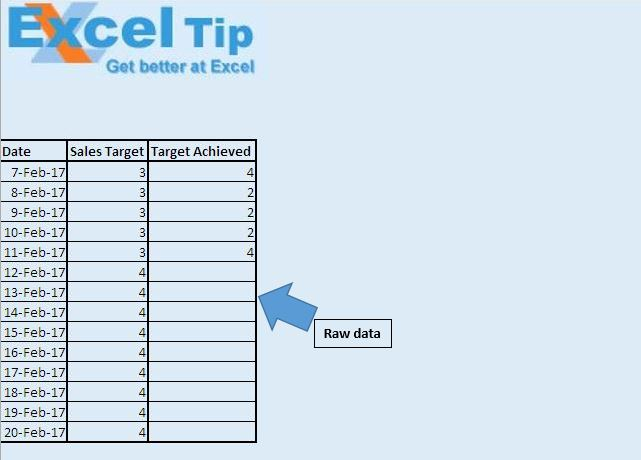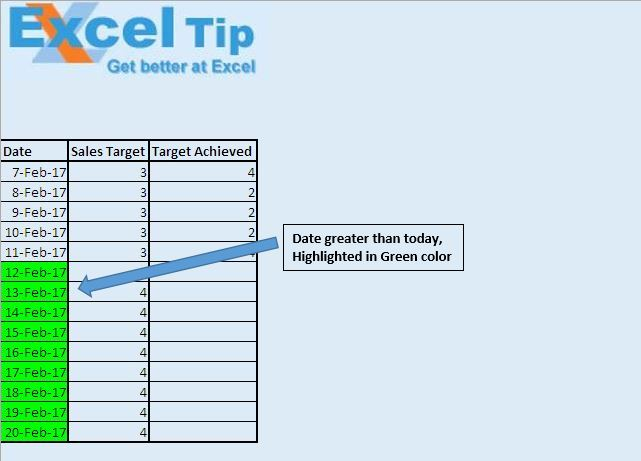# Change font color based on Date using VBA in Microsoft Excel

In this article, we will use workbook event to highlight dates greater than the present date.

Raw data for this example consists of daily sales target and target achieved.We want to highlight days which are greater than the present date.Logic explanation

SetColor” macro is used to highlight dates which are greater than the present date.

ResetColor” macro is used to reset formatting of specified source.

We have used two workbook events, workbook open event and workbook before close event.

Workbook open event is fired when workbook is opened. On opening the workbook, it will automatically run the “SetColor” macro.

Workbook before close event is used to reset event associated with the workbook open event.

Code explanation

If IsDate(ActiveCell.Value) And ActiveCell.Value > Date Then

The above code is used to check whether value in the cell is of date data type and is greater than the present date.

ActiveCell.Interior.Color = RGB(0, 255, 0)

The above code is used to assign green color to the active cell.

Set Source = Range("A1", Range("A1").SpecialCells(xlCellTypeLastCell))

The above code is used to select all the data within the excel sheet.

```Option Explicit

Sub SetColor()

If IsDate(ActiveCell.Value) And ActiveCell.Value > Date Then
ActiveCell.Interior.Color = RGB(0, 255, 0)
Else
ActiveCell.Interior.Color = RGB(221, 235, 247)
End If

End Sub

Sub ResetColor()

'Declaring variables
Dim Rng, Source As Range
Dim IntRow As Integer, IntCol As Integer

'Specifying all the cells as source range
Set Source = Range("A1", Range("A1").SpecialCells(xlCellTypeLastCell))

'Looping through all the cells
For Each Rng In Source
'Checking whether cell contains a value
If Not IsEmpty(Rng) Then
'Checking whether cell contain value of date data type
If IsDate(Rng.Value) Then
Rng.Select

'Assigning Green color if value is greater than today date
If DateValue(Rng.Value) > Date Then
ActiveCell.Interior.Color = RGB(0, 255, 0)
Else
ActiveCell.Interior.Color = RGB(221, 235, 247)
End If
End If
End If
Next Rng

End Sub

'Insert below code in ThisWorkbook module

Option Explicit

Private Sub Workbook_Open()

With Worksheets("Main")

'Event fired on entry to worksheet
.OnEntry = "SetColor"
'Event fired on sheet activation
.OnSheetActivate = "ResetColor"

End With

End Sub

Private Sub Workbook_BeforeClose(Cancel As Boolean)

With Worksheets("Main")
.OnEntry = ""
.OnSheetActivate = ""
End With

End Sub
```